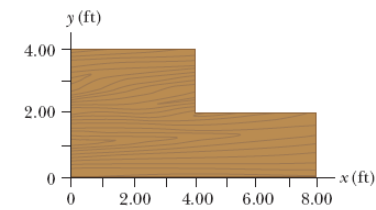# Problem: Find the x- and y-coordinates of the center of gravity of a 4.00-ft by 8.00-ft uniform sheet of plywood with the upper right quadrant removed as shown in the figure below. Hint: The mass of any segment of the plywood sheet is proportional to the area of that segment.x=_____y=_____

###### FREE Expert Solution

X-coordinate of the center of mass:

$\overline{){{\mathbf{x}}}_{\mathbf{c}\mathbf{m}}{\mathbf{=}}\frac{{\mathbf{m}}_{\mathbf{1}}{\mathbf{x}}_{\mathbf{1}}\mathbf{+}{\mathbf{m}}_{\mathbf{2}}{\mathbf{x}}_{\mathbf{2}}\mathbf{+}\mathbf{.}\mathbf{.}\mathbf{.}\mathbf{+}{\mathbf{m}}_{\mathbf{n}}{\mathbf{x}}_{\mathbf{n}}}{{\mathbf{m}}_{\mathbf{1}}\mathbf{+}{\mathbf{m}}_{\mathbf{2}}\mathbf{+}\mathbf{.}\mathbf{.}\mathbf{.}\mathbf{+}{\mathbf{m}}_{\mathbf{n}}}}$

Y-coordinate of the center of mass:

$\overline{){{\mathbf{Y}}}_{{\mathbf{cm}}}{\mathbf{=}}\frac{{\mathbf{m}}_{\mathbf{1}}{\mathbf{y}}_{\mathbf{1}}\mathbf{+}{\mathbf{m}}_{\mathbf{2}}{\mathbf{y}}_{\mathbf{2}}\mathbf{+}\mathbf{.}\mathbf{.}\mathbf{.}\mathbf{+}{\mathbf{m}}_{\mathbf{n}}{\mathbf{y}}_{\mathbf{n}}}{{\mathbf{m}}_{\mathbf{1}}\mathbf{+}{\mathbf{m}}_{\mathbf{2}}\mathbf{+}\mathbf{.}\mathbf{.}\mathbf{.}\mathbf{+}{\mathbf{m}}_{\mathbf{n}}}}$

The block has a missing quarter. The mass of the block is unknown

The statement, "The mass of any segment of the plywood sheet is proportional to the area of that segment" implies that we can use the area of each piece instead of its mass.

Let's begin with the x-coordinate of the center of mass:

84% (162 ratings)###### Problem DetailsFind the x- and y-coordinates of the center of gravity of a 4.00-ft by 8.00-ft uniform sheet of plywood with the upper right quadrant removed as shown in the figure below. Hint: The mass of any segment of the plywood sheet is proportional to the area of that segment.

x=_____
y=_____